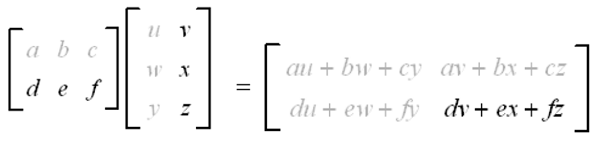# numpy.matmul() for Matrix Multiplication | Linear Algebra using Python

Linear Algebra using Python | numpy.matmul() for Matrix Multiplication: Here, we are going to learn about the numpy.matmul() for matrix multiplication in Python?
Submitted by Anuj Singh, on May 22, 2020

Prerequisite: Linear Algebra | Defining a Matrix

Numpy is the library of function that helps to construct or manipulate matrices and vectors. The function numpy.matmul() is a function used for matrix multiplication. The example of matrix multiplication is shown in the figure.

There is a fundamental rule followed by every matrix multiplication, If the matrix A (with dimension MxN) is multiplied by matrix B (with dimensions NxP) then the resultant matrix (AxB or AB) has dimension MxP. In other words, the number of columns in matrix A and the number of rows in matrix B must be equal.Syntax:

```    matrix_Multiplication = numpy.matmul(Matrix_1, Matrix_2)
```

Input parameters: Matrix_1, Matrix_2 the two matrices (following the above-mentioned rule).

## Python code to demonstrate example of numpy.matmul() for matrix multiplication

```# Linear Algebra Learning Sequence
# Matrix Multiplication using
# function in numpy library

import numpy as np

# Defining two matrices
V1 = np.array([[1,2,3],[2,3,5],[3,6,8],[323,623,823]])
V2 = np.array([[965,2413,78],[223,356,500],[312,66,78]])

print("--Matrix A--\n", V1)
print("\n\n--Matrix B--\n", V2)

# using function np.matmul( )
AB = np.matmul(V1, V2)

print("\n\n--Matrix Multiplication AB-- \n", AB)
```

Output:

```--Matrix A--
[[  1   2   3]
[  2   3   5]
[  3   6   8]
[323 623 823]]

--Matrix B--
[[ 965 2413   78]
[ 223  356  500]
[ 312   66   78]]

--Matrix Multiplication AB--
[[   2347    3323    1312]
[   4159    6224    2046]
[   6729    9903    3858]
[ 707400 1055505  400888]]
```

Preparation

What's New

Top Interview Coding Problems/Challenges!